# 回文树介绍(Palindromic Tree)

### 回文树的结构

#### 节点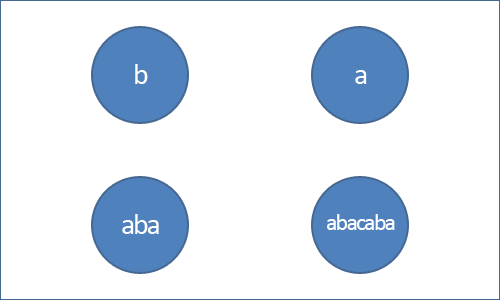#### 边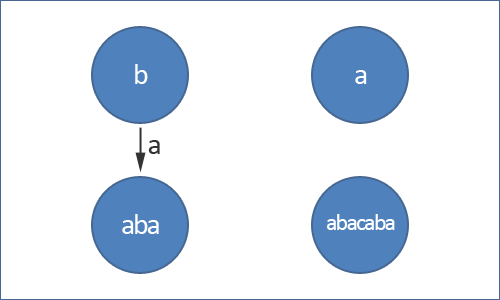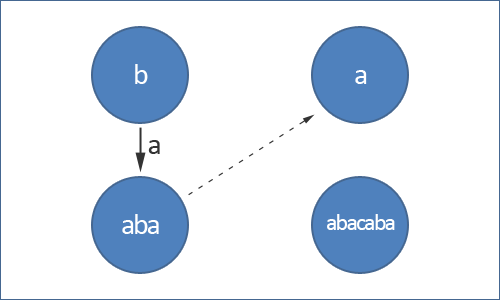“回文树”这个名字可能会让人产生疑惑，因为回文树这个数据结构并不是一棵普通的树，它有两个根，一个根表示长度为-1的回文串，是我们为了方便操作加进去的，长度为1的回文串可以通过它左右两侧各添加一个字符得到。另一个根表示长度为0的回文串，即空串。

### 回文树的构造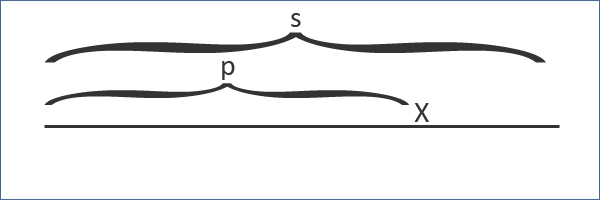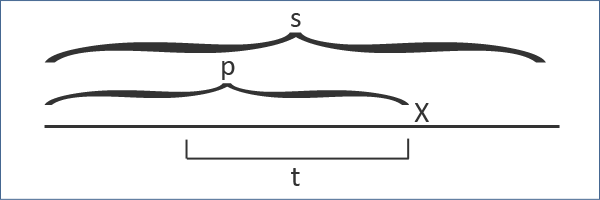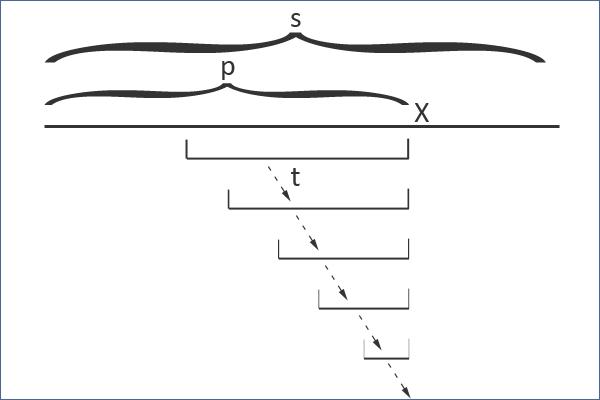t$t$出发的后缀链接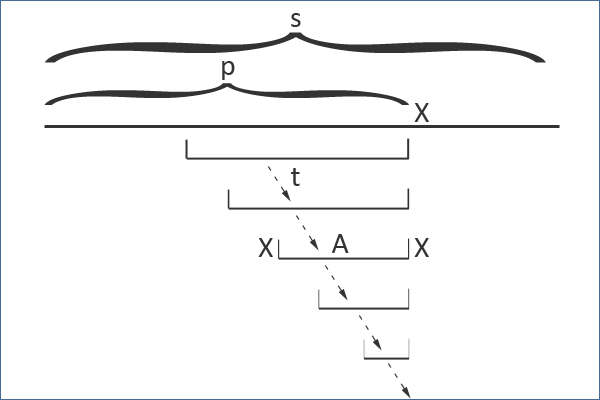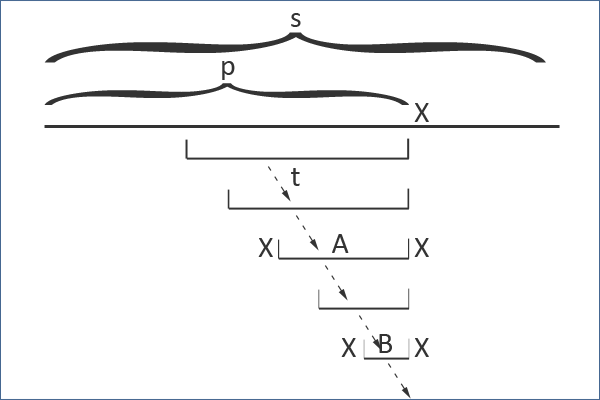#include <bits/stdc++.h>
using namespace std;

const int MAXN = 1005;

struct node {
int next;
int len;
int num;
};

int len;
char s[MAXN];
node tree[MAXN];
int num;            // node 1 - root with len -1, node 2 - root with len 0
int suff;           // max suffix palindrome
long long ans;

int cur = suff, curlen = 0;
int let = s[pos] - 'a';

while (true) {
curlen = tree[cur].len;
if (pos - 1 - curlen >= 0 && s[pos - 1 - curlen] == s[pos])
break;
}
if (tree[cur].next[let]) {
suff = tree[cur].next[let];
return false;
}

num++;
suff = num;
tree[num].len = tree[cur].len + 2;
tree[cur].next[let] = num;

if (tree[num].len == 1) {
tree[num].num = 1;
return true;
}

while (true) {
curlen = tree[cur].len;
if (pos - 1 - curlen >= 0 && s[pos - 1 - curlen] == s[pos]) {
break;
}
}
return true;
}

void initTree() {
num = 2; suff = 2;
tree.len = -1; tree.sufflink = 1;
tree.len = 0; tree.sufflink = 1;
}

int main() {
scanf("%s", s);
len = strlen(s);

initTree();

for (int i = 0; i < len; i++) {
ans += tree[suff].num;
}
cout << ans << endl;
return 0;
}

### 结论

©️2019 CSDN 皮肤主题: 大白 设计师: CSDN官方博客